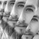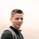# Relative Strength Volatility Variable Bands [DW]จำนวนเข้าชม 5611
This is an experimental adaptive trend following study inspired by Giorgos Siligardos's Reverse Engineering RSI and Tushar S. Chande's Variable Moving Average.

In this study, reverse engineered RSI levels are calculated and used to generate a volatility index for VMA calculation.

First, price levels are calculated for when RSI will equal 70 and 30. The difference between the levels is taken and normalized to create the volatility index.

Next, an initial VMA is calculated using the created volatility index. The moving average is an exponential calculation that adjusts the sampling length as volatility changes.

Then, upper and lower VMAs are calculated by taking a VMA of prices above and below the initial VMA. The midline is produced by taking the median of the upper and lower VMAs.

Lastly, the band levels are calculated by multiplying the distance from the midline to the upper and lower VMAs by 1, 2, 3, 4, and 5.

Bar colors are included. They're based on the midline trend and price action relative to the upper and lower VMAs.

-> Migrated to v4.
-> Stability Fix: Added correction for NaN values to stabilize initial states.
-> Reorganized script structure
-> Updated style theme.
สคริปต์โอเพนซอร์ซ

จากใจ TradingView ผู้เขียนสคริปต์นี้ได้เผยแพร่เป็นโอเพนซอร์ส เพื่อให้ผู้ค้าสามารถเข้าใจและตรวจสอบได้ ยินดีด้วย! คุณสามารถใช้มันได้ฟรี แต่การใช้โค๊ดนี้ซ้ำเพื่อการเผยเเพร่ อยู่ภายใต้ กฎของเรา คุณสามารถตั้งเป็นรายการโปรดเพื่อใช้กับชาร์ตได้

ต้องการที่จะใช้สคริปต์นี้บนชาร์ตใช่ไหม?

## ความคิดเห็นwhat would be the rules to trade this?
For example: to trade pullbacks -> If midline depicts downtrend (red) we could short when price touches midline and exit when price touches the lower band? viceversa for uptrend.
What do you suggest? It'd be great to see a backtest strategy too!